## ACID BASE REACTIONS

#### Introduction

The concept 'acid' / 'sour' we all know from taste of lemons, vinagre or sour apples. It is a gererally known word, used for ages and intruded in chemistry.
But be carefull please. In chemistry only a few acids can or may be tried, tasted in that way. Never taste a chemical substance!!
This can be very dangerous. Such an acid can be poison or so strong that it immediatly reacts with other substances in your mouth, in your stomach. It can burn.

At the other hand, we have as an opposite of acid: the 'base'.
In chemistry acids and bases are each others opposite, but what does that mean in practice? Is there also a kind of base-taste? Do we have daily things that ar basic, where others are acid?

Indeed. Some people know the taste of soap, for example. That's one of the substances with a basic character, just like soda (=sodium carbonate)

The definitions of acid and base are various and are different from the old definitions. The most well known definition is based on the concept of protons (=H+)

No longer we call a base the substance that can provide OH--ions, as we did in the old days. Some study books may still have this old definition.

Question 1
"The neutralisation of diluted cloric acid with a solution of sodium hydroxyde can be represented as follows:
H+(aq) + OH-(aq)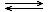H2O(l)
Antwoord 09-01

Question 2
Give an electronic structure (as complete as possible) of the atom of H and of the ions H+, H-, OH- and (H3O+).

Question 3
Explain why also the word 'protondonor' is used for an acid.

#### Content

 1.3 Various bases 2.4 KA (acid constant) and KB (base constant) 3.2 pH 4. pH measuring 5. Salts and Electrolyts 6. Buffer solutions 7. Acid base character and the periodic table 8.2 Calculations with KA and KB and with pKA and pKB 9.1 Acid base Indicators

## 1. Definitions

Definition according to Brønsted:

ACID:
any substance that can donate H+-ions

BASE:
any substance that can accept H+-ions

Definition according to Lewis:

ACID:
any substance that can suffer increase of, has room for free electron pairs.
or: a structure that suffers lack of (free) electron pairs.

BASE:
any substance that contains (more than) enough free electron pairs, and that can put available those pairs.

Question 4
Explain the character of the particles HCl and NH3 using the above definitions.

Question 5
Starting with the following data, you must try to explain why teeths are more resistant against acids after treatment with fluor(ide).
the data are:
• the main components of bone in teeths is hydroxy-apatite: Ca(OH)2.3Ca3(PO4)2
• an ion OH- can easily been substituted by an ion F- (charge and size are more or less the same.).
That's how is made the substance fluor-apatite: CaF2.3Ca3(PO4)2
• The optimal percentage of OH- in apatite is 2%
• Tap water can be fluoridised with NaF
• F- is a weaker base than OH-

#### Neutralise

Question 6
A solution of 25oC with pH = 7
1. does not contain salts
2. contains equal amounts of H+ and OH-
3. does not contain H+ and no OH-
4. does not contain acid or base

If acids react with bases, we call that 'neutralisation' (at real neutralisation we add equal (equivalent) amounts of both toghether).
Neutralisation is is always an acid base reaction with transfer of protons (ions H+).

#### Protons are not free available.

They always are connected to one or another particle. Yes, they can be transferred, but not at distance. Only when acid and base touch each other, transfer of a proton is possible.

So acids donate H+. We can conclude that, but this phenomena has a cause. Why is it that acids donate Hydrogen ions? How to discover that any structure has such an option of donating H+?

Question 7
1. Will the following substance be an adic or not? Or: is any particle capable to donate protons?
2. Try to include in your answer the polar character of the bondings in the molecule.
 CH4 H2S NH3 H2O HCOOH HCl HCN H     | H - C - H     |     H H   /  S   \    H H   H  \ /   N   |   H H  \   O   |   H OH      / H - C = 0 H - Cl H - C ≡ N

The H+-ion can appear from a strong polar bond with Hδ+-atoms in it; condition is that within the structure are enough repelling forces between that Hδ+ and another positive charged close atom.
non polar bonds normally do not participate in acid base reactions.

### 1.2 Various types of acids

1. Organic acids
We know lots of organic acids; the most important for this course are those with a carboxyl group (the alkanoic acids). Phenol is another kind of organic acid.

We will talk about carboxylic acids, amino acids, fatty acids and phenol, but also about bases.
Here only some questions

Question 8
consider the structure of a carboxylic group. What could be the cause of the acid character of this group?

Question 9
The carboxylic group is not a very strong acid.
Rather strong are methanoic acid and trichloromethanoic acid. Comparing those two, the last one turns out to be stronger then the first one.
Explain that phenomena.

Phenol (benzene with a hydroxyl group -OH) is a liquid with a seak acid character.
Hydroxyl groups normally are found in alcohols and have no acid character at all. But the one of Phenol has, is capable to donate H+ ions from the hydroxyl group.
This remarkable fact exists thanks to the presence of that benzene ring (with the six free electrons in the ring). The absence of a proton, and so the presence of O- at the ring, will strenghten the degree of freedom of those six electrons (+1). Seven free electrons make the structure even more stable than it already was.
Losing H+ increases the stability of the structure; and that creates the (weak) acid character.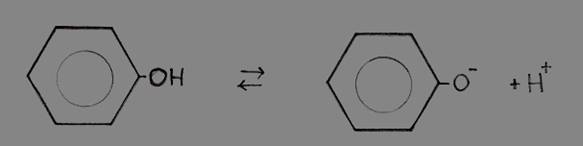or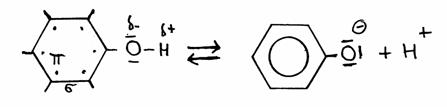Phenolphenolate + H+

The extra electron of the phenolate will participate in the free movement of the six electrons in the ring, and stabilize the phenolate.

2. Oxy-acids; Oxygen containing acids
The oxy-acids can be made - in general - from the corresponding oxyde with water.

Example: P2O5 + 3H2O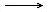2H3PO4

Att.: We talk now about reactions wherein the oxidation number does not change.

Question 10
Give the reaction equations of the production of the following Oxygen containing acids: sulphuric acid, carbonic acid, hyper chloric acid, permanganese acid.

Most of these oxydes come from the non metals (although not all!!).

3. Hydrogen acids (without Oxygen)
They are produced directly from an element (mostly a non metal) with Hydrogen.
The best known example is Chlorine with Hydrogen: Cl2(g)+ H2(g)2HCl(g)

A special example is: HCN

Question 11
Looking at the non metals, we come to the following Hydrogen acids: HCl, HBr, HI, H2S, HF.
Att.: there are more bondings between Hydrogen and the non metals, like H2O and NH3.
Explain the character of these substances, considering their acid or basic character.

4. Cationic acids
Certain polyvalent positive ions (2+ and 3+) have the property to attract water molecules. We say: they are hydrated.
There is a strong attraction to the negative charge (δ-) of those water molecules with their charge δ+ also close to those strong positive metal ions.
You must understand that this will cause repulsion: maybe protons will be repelled, donated.

#### cationic acids (= positive metal ion) with watergeconjugated base + hydronium ion.

Thats how appears the possibility to donate H+.

Here we deal with a number of polyvalent positive metal ions, like Al3+, Cu2+, Fe2+ or Fe3+, and more.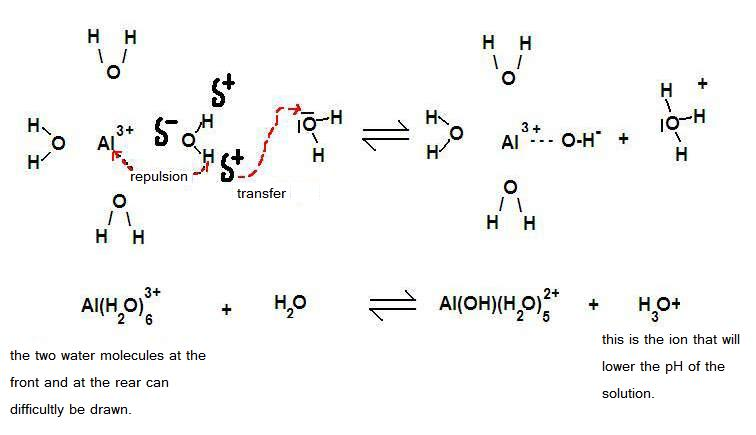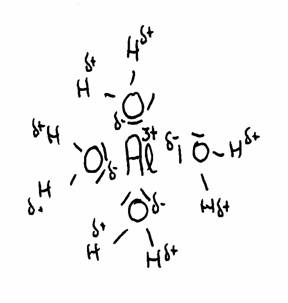Question 12
Makes that sense?: Cationic acids are - in general - solutions with polyvalent positive metal ions.

Question 13
A special case of such a positive ion with an acid character is the ammonia ion:

NH4+ + H2ONH3+ H3O+
1. Explain to which side this equilibrium will shift.
2. Why?
3. What is the consequence in practice?

5. Negative ions
Question 14
Negative ions - normally - can be basic, or: they can catch protons.
Explain that negative ions normally do not act as acids.

But still! There are negative ions that can act as acids, and donate H+.
Example: HCO3-.
This example shows that we deal now with amphoteric particles.
This type of negative ions still contain more Hydrogen; they come from polyprotonic acids that have not yet donated all H+.
You find themin the table with acids and bases in both colums.
Check that.

### 1.3 Different types of chemical bases

In module 04 the different types of bases were already named:
1. Organic molecules
Normally those organic substances that contain Nitrogen where that Nitrogen atom contains a free electron pair.
Think of amines and the amino acids.

Question 15
Give the reaction equation in ionic formulas of the reaction with an acid with:
1. amino-ethane;
2. Alanine.

Question 16
Give an organic substance that also is a diprotonic base.

2. Negative ions
In principle all negative ions can catch, take up H+ ions, so they are bases, wether weak or strong.
Some are exstremely weak, like Cl-. Then the basic character is only theory. In reality this is nothing.

Question 17
Put in order of increasing basicity the following particles (using the table of acids and bases):

Br-     0H-     HCO3-     H2PO4-     CH3COO-     CH2ClCOO-     SO42-     HSO4-

3. Some positive ions
Cathions normally are acids, as we have seen. But if they are polyprotonic, polyvalent, so they can donate more than one proton, then we are close to this possibility:
For example, if the 3+ hydrated Aluminium ion (Al(H2O)63+) has donated one proton, then Al(OH)(H2O)52+) was formed.
This is a positive ion that is able to take back that proton, and thus react as a base. The same particle can of course also continue with donating (and form Al(OH)2(H2O)4+ ).
After all the particle can donate as well as capture, so it is amphoteric.

 Al(H2O)6 + H2OAl(OH)(H20)5- + H3O+ acid base base acid

Question 18
1. In the above equation, indicate why substances are acid of base as given;
2. and explain at which side we find the strong and the weak substances.

4. Neutral molecules
Like, for example: ammonia and water (where water is amphoteric)

Question 19
If the two gases ammonia(g) and hydrogenchloride(g) meet (and greet), then a white smoke is produced.
1. Give the reaction equation in molecular formulas
2. Explain why this reaction is an acid base reaction.

## 2. The acid base equilibrium; conjugated pairs.

Question 20
Given the following equilibrium: H2O + H2OH3O+ + OH-
1. Explain why this equilibrium can only exist in aqueous environment
2. At what side are the strong and the weak substances?
3. Which is the value of the equilibrium constant?

### 2.1 Kw and the autoprotolysis of water.

In an aqueous environment there are incredible amounts of water molecules that continuously collide with each other. The water molecule has the (very weak) tendency to donate protons, but also to take protons (so water is amphoteric)
During collisions of two water molecules, the following can happen:

 H2O + H2OH3O+ + OH- ΔH > 0 weak base weak acid strong acid strong base

Att.: the two ions are produced in equal amounts.
The water equilibrium only can be realised in aqueous environment, and is dislocated very much to one side, and has an equilibrium constant: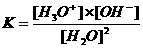→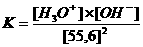→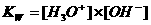In neutral aqueous solutions with temperature of 25ºC the following will happen:
Of each mol water molecules (about 0,6 x 1023) "only" 6 x 1016 water molecules will effectively catch a proton and the same number of 6 x 1016 water molecules will donate.

In water of 25ºC the concentration [H3O+] = [OH-] = 10-7 mol/dm3   or: pH = pOH = 7

Kw (autoprotolysis constant) = K x (55.6)2 = [H3O+] x [OH-] = 10-14 mol2/l2

That autoprotolysis of water is an endothermic process.
At high temperatures more ions are produced, their concentrations increase (for example: 10-6 mol/dm3 instead of 10-7 mol/dm3).
The value of Kw will change in this case from 10-14 to 10-12.

Question 21
What will be - more or less - the value of K w in ice?

Question 22
Given: In boiling water the value of the pH is not equal to 7.
Statement: Boiling water still is a neutral substance.
True of false?

### 2.2 Conjugated pairs

Question 23
What does it mean?: HA and A- form a conjugated pair/couple.

Let's indicate an acid with HA.
Dissolved in water, HA can split off / donate H+.

In the same way we call the A- a base,
that - dissolved in water - can take up H+.

The following equilibria will be established:

acid solutions:
HA + H2OH3O+ + A-
acid  base         acid     base
A- is the geconjugated base of the acid HA
[water acts as a base]

basic solutions
A- + H2OHA + OH-
base acid         acid     base
HA the geconjugated acid of the base A-
[water acts as an acid]

An acid always turns into a base (and a base always becomes an acid).
If the difference between those two is not more than one proton (H+), such a couple is called a geconjugated pair.

Table I contains a table with acids and bases, in all cases accompanied by their conjugated partner.

Question 24
• HA + H 2H3O+ + A-
• A- + H2OHA + OH-
In the above two equations, various conjugated paires can be discovered.
Which ones?

### 2.3 Weak and strong

An acid base reaction, in principle, will always be a chemical equilibrium, often with very strong substances at one side and weak substances at the other side of the arrows.
The equilibrium dislocates/shifts always to the side of the weak substances, and those weak substances 'survive'.

Question 25
Use table I with the acids and bases, and explain why the following substances yes or no will react:
1. Hydrogen Iodide with water
2. Hydrogen sulfide with fluoride
3. Hydrogen carbonate with phosphate
4. hydrated Aluminium ions with phosphate
5. sulfuruc acid with carbonate
6. the ammonium ion with the ion hydroxyde

The very simplified rule:
When in the acid base table an acid is higher located than the base, a reaction will take place.
Behind this rule is of course a reason: stronger acids react with stronger bases and produce weaker bases and weaker acids. In the table the stronger acids are positioned more up, and the stronger bases more down.

### 2.4 KA (acid constant) and KB (base constant)

 acid solutions HA + H2OH3O+ + A- acid base acid base A- is conjugated base of the acid HA KA = acid constantIf KA is very large (or pKA is very small), HA is a very strong acid An equilibriium always will shift to the side of the weaker ones.

 basic solutions A- + H2OHA + OH- base acid acid base HA is conjugated acid of the base A- KB = base constantIf KB is very large (or pKB is very small), A- is a very strong base An equilibriium always will shift to the side of the weaker ones.

Question 26
Study the table with conjugated acid base couples, and try to confirm the following statements:
1. when KA is very large (or pKA very small), then HA is a strong acid
2. polyprotonic acids can donate more than one proton, for example H3PO4
3. polyprotonic bases can take more than one proton, for example CO32-
4. amphoteric particles can react as acid as well as base, for example HPO42-

Question 27
Explain if the following salts possibly have the opportunity to donate or to catch protons:
Sodium carbonate and Calcium Hydrogen carbonate.

## 3. The p-values

### 3.1 pKA and pKB

mathematically:            p...... = -log .......

KA and KB are the acid / base constants, who's values can be find in tables.
Often the values of concentrations (certainly the mathematical products of concentrations) are extremely small, like for example: 10-6 mol/l.
Therefore were introduced the so called p-values; they make writing of and calculating with concentrations a lot easier.

KA and KB also often have very small values, like: 10-12 or 10-7
In chemical calculations and in the books we use the p-values 12 or 7

Question 28
In aqueous neutral solutions with a temperature of 25ºC:
• data: [H3O+] = [OH-] = 10-7 mol/dm3       i.e.;     pH = pOH = 7
• Kw (constant of the autoprotolysis) = K x (55.6)2 = [H3O+] x [OH-] = 10-14

Prove the following statement: pKw = pH + pOH

pKW at 25ºC has a value of ±14; at 100ºC that value is ±12

Question 29
Fill in the empty places, via calculations and by using the table:

 concentration value of KA or KB value of the pH of the solution A 0.1M HCl B 0.1M HAc C 0.3M HN3 D 1M Na2CO3

Question 30
Use the acid base table and check the product of KA x KB and the sum of pKA + pKB of the conjugated couples.
1. What do you observe, and what is your conclusion?

### 3.2 pH

Study the following scheme; try to understand it and to explain:

 pH -1 0 1 2 3 4 5 6 7 8 9 10 11 12 13 14 15 pOH 15 14 13 12 11 10 9 8 7 6 5 4 3 2 1 0 -1 very strong and/or concentrated acid solutions acid solutions neutral solutions basic solutions very strong and/or concentrated basic solutions

## 4. Measuring pH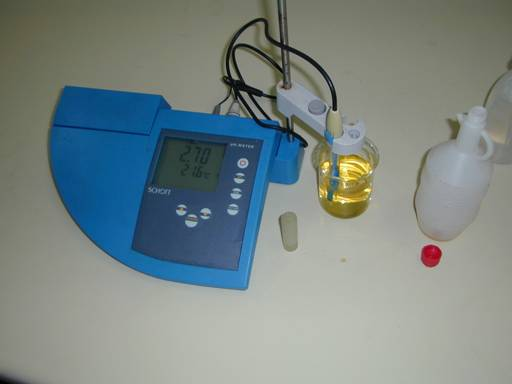Study carefully the picture.

The pH-meter (blue) shows a valueof 2.7: here we've got a vinegar solution (the yellow liquid in the beaker).
Next to the solution is the bottle with the rest of the vinegar (almost empty).
The small plastic tube close to the pH-meter is a protection cover of the electrode.
The electrode itself is immerged into the vinegar (normally the electrode is kept in that cover).

Question 31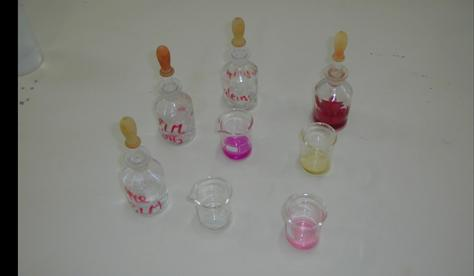This photo can be converted into a table with three columns and three rows.
Control the following table; is it right?

 phenolphtaleene methyl red ammonia(aq) carmin red yellow HCl(aq) colorless red

#### Indicators are weak acids with weak conjugated bases (present in equilibrium).

The structure of an indicator mostly is an organic and rather complex molecule.
Very often we use the short formula: HIn.
That H is of course the proton that can be donated (the indicator being a weak acid) and the rest - In - is the biggest and complex part of the molecule.

In module 8 we already used an example of a substance that has the opportunity to visualise the location of the equilibrium. That was indeed an indicator.
The acid base indicator is applicable as such because HIn has another color than In-.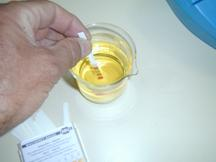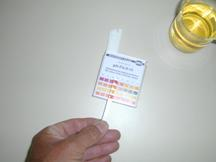We measure the pH-value of vinegar with universal indicator paper. One paper strip is hold for a moment in the vinegar.
Four indicators on the strip get their own color each, and the combination of those four must be compared with the standard colors on the box.
In this case the four colors correspond with a pH of about 3 (not easy to see on the picture)

Besides the universal indicator paper their are various other types of indicator paper.
Sometimes the colorants are completely vegetable.

Question 32
What plant do you know with this property?

Question 33
A certain solution shows different colors with indicators:
Litmus becomes blue. Bromo thymol blue also becomes blue, and phenolphtaleine becomes colorless.
Explain what will be, more or less, the pH of this solution.

## 5. Salts and electrolytes as acid or base

When substances are composed of ions, we often call them electrolytes.
The word has to do with the property of ionic substances (there are charged particles present!) to conduct electrical current (see module 5), at least when those particles can move freely (are not hold in an ionic lattice).
Electrolytes are salts in the first place, but also other substances with ions, like acids and bases (certain when they contain OH-), belong to the electrolytes.

Question 34
Is the following statement true or false?: a substance composed of ions (exceptions H+ and OH-) is a salt.

If a reaction takes place with ionic substances (substances with ions), then you can write the reaction equation in ionic formulas, but also in empiric formulas. We prefer here the ionic formulas.
Only when you must execute calculations on basis of the reaction equation, then it is better to work with empiric formulas. Example: In that case do not use Na+ and Cl-, but NaCl.

Example:
HCl(aq) + NaOH(aq) → NaCl(aq) + H2O(l)         empiric formulas

H3O+ + OH- → H2O(l)         ionic formulas

The two equations represent exactly the same process.

The substances built up of ions, carry the character of those ions; you must consider any ion.
An ion has a neutral or an acid or a basic character. The dominant character determines the final character of the salt as a whole.
In the case of NaCl, built up of the ions Na+ and Cl- not any of the ions has an acid of basic character. The salt as a whole must be a neutral substance; does not influence the pH of its solution.

But many other salts do have that influence: like Sodium carbonate, built up of ions Na+ and CO32-. Analysis shows that the first ion is neutral, but the second is rather basic.
A solution of this salt wil have a pH above 7. The solution will be basic.

Copper(II)sulfate contains ions of sulfate (very weak basic) and ions of Copper(II) (rather acid). In a solution of this copper sulfate, the acid character will dominate.
Copper(II)sulfate will cause in the solution a pH lower then 7.

Concluding: You must consider any ion apart, and analyse and compare.

Examples:
Use table I
We have a solution of Sodium Hydrogen carbonate in water. Let's analyse this solution:
First you must know that all salts with Sodium ions are soluble in water. Our salt will dissociate in ions:
Na+ and HCO3-; these ions remain hydrated in water.
Na+ with its charge of +1 will have no influence on the water molecules, does not cause any formation of protons and does not influence the pH of the water.
HCO3- is another story: The table shows a KA = 10-10 and a KB of 10-8
KA (10-10) < KB (10-8), so, HCO3- has an amphoteric character, where the basic character dominates, because KB > KA.
The ion remains in the water with a basic character, and the solution of the salt NaHCO3 in water is not neutral, but will have a pH above 7 (somewhere between 8 and 10, depending on the amount of salt).
In this way you must be able to analyse any (salt)solution in water.

Connected to this topic, it remains important to check the solubility of the salt. The active ions must really be present in the solution for influencing the pH in that solution.
A very bad soluble salt does not deliver enough ions to have direct influence. Apart from the table with acids and bases, you must also consult the table with solubility of salts to make sense.

Question 35
Of the following solutions you must explain the acid, basic or neutral character, comparing and using ionic formulas and ionic equations.
NaBr CuCl2   K3PO4   Ca(OH)2   Ba3(PO4)2   Na2HPO4   Al2(SO4)3   CuS   HCl   CH3COONa   CaCO3
Make a table that contains all these salts, the ions, the character (acid or basic) that dominates, an estimation of the pH of the solution.

## 6. Buffer solutions

Question 36
Calculate the pH-values of 1M HAc, and then the pH-values of a mixture of 1M HAc + 1M NaAc

In a solution of a weak acid in water the rule is: [H3O+] = [A-] (These two appear in equal amounts from HA).
But note: This is not the case in a buffer solution where there is an excess of Ac-.
In a buffer we say: [H3O+] ≠ [A-]. A buffer solution contains not only the weak acid, but also a salt of that weak acid.

Adding a bit of a strong acid to a buffer solution (that means some extra hydronium or oxonium ion H3O+ ), then immediately that extra added acid will be neutralised by the large amount of in the buffer present weak base (Ac-).
The following reaction takes place:

Ac-(from the buffer) + H3O+(from the added strong acid)HAc + H2O

NB: [Ac-] decreases a littlebit and [HAc] increases a bit.

Adding a bit of a strong base, for example OH-) to a buffer, that extra base will immediately be neutralised by the large amount of weak acid HA in that buffer.
The following reaction will take place:

HAc(from the buffer) + OH-(from the added strong base)Ac- + H2O

NB: [Ac-] increases a littlebit and [HAc] will decrease a bit.

two possible definitions of the buffer:

#### An aqueous solution: in which the pH hardly will change, even with adding acids or bases. containing a mixture of a weak acid + its weak conjugated base, both in considerable concentrations.

The Buffer formula: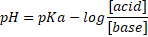or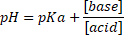A 'buffer' of good quality will always contain more or less equal amounts of the (weak) acid and of the (weak conjugated) base.

Question 37
1. Are the following statements I and II true of false? Explain your choice.
1. To get a good buffer solution, you always must chose an acid with pKA close to de desired pH.
2. Out of the two acid base couples: HCO3- / CO32- and H2CO3/HCO3-, the first fits best as a buffer in human blood.
2. Calculate the pH of a mixture of 1 mol Sodium oxalate...
1. in 2 liter of water
2. + 1 mol Sodium Hydrogenoxalate in 2 liter of water.
3. Answer the next question: A mixture of kitchen salt and hydrochloric acid will be a buffer?

When the pH of the blood (7.3) changes, even if it is just a littlebit, this can be mortal. A human accepts nor survives a real pH-change in blood. Yet the blood has te transport acids and bases like carbon dioxyde, lactic acid, amino acids, phosphates and more. They may not change the pH of the blood.
How the body will manage that? What mechanism has the blood to prevent changes?
Of course blood contains the necessary buffers, containing:
• the groups -NH2 (amino, BASE) and -COOH (carboxyl group, ACID)
• groups present in proteins, blood proteins
• the ions carbonate and bicarbonate
• various phosphates

Buffer solutions always function in such a way that the buffer contains weak acids and bases.

Question 38
1. We have 500 mls solution at 25 degrees Celcius of0.1M NaAc (Sodium acetate). Adding 2 mls 2M HCl will decrease immediately the Ph from 8.87 to 3.68
2. The same addition to a 500 mls buffer of 0,1M HAc + 0,1M NaAc only causes a pH decrease from 4.74 to 4.68
3. Adding to the same buffer not acid, but 2 mls 2M NaOH, causes only a very small pH-increase.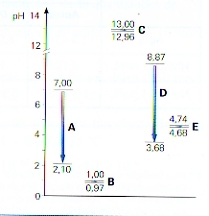In the figure you see such changes: five times 2 mls 2M HCl is added to 500 mls...
1. pure water
2. a solution of 0.1M strong acid
3. a solution of 0.1M strong base
4. a solution of 0.1M weak base
5. a buffer
1. Controll the statements in I and II (see above) by calculating with the buffer formula.
2. Calculate the pH-change in III, at adding 2 ml 2M NaOH to 500 ml buffer 1MHAc/Ac
3. Controll and explain the data in the diagramme.

If blood must transport an acid, then any basic ion/molecule like an amino group can be responsible for that. When a base must be transported, an acid ion/molecule will be responsible.

Other locations in the human body with this kind of regulations:
• in urine: the pH can vary between 5 and 8, or, urine must be close to neutral.
• in the digestion system: different enzymes take care for a good digestion, and each of them will have an own preference for pH.

 where? which enzyms? the values In the stomach peptase, rennase en lipase 1.5 - 4 In the bowels maltase, saccharase, lactase, ereptase 6.6 - 8.5

## 7. How the acid base character is connected to the position in the Periodic Table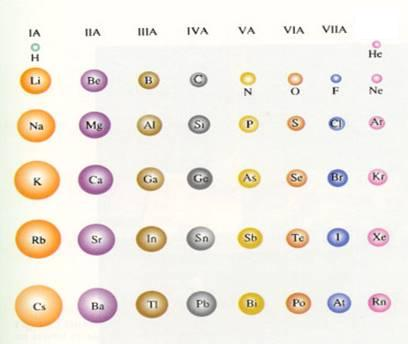Study well this periodic tabel and lets have a special look at some periods.
(the noble gase are left out; they do not form any bondings, also no acids or bases)

 I. Period 2, Li - F Compounds with Hydrogen (hydrides) LiH       BeH2       BH3       {CH4}         NH3         H2O         HF Strong Base ---------------------weak base - neutral - weak acid From left to right we see a gradual transition from base to acid, where water is a real neutral substance, also CH4 for other reasons than water. The Hydride ion (H-) is is very basic and can be donated by LiH and BeH2. BH3 and NH3 have the tendency to catch H+, so they are weak basic, where NH3 is weaker than BH3. Compounds with Hydrogen and Oxygen LiOH     Be(OH)2     B(OH)3 = H3BO3     H2CO3 (=H4CO4)     HNO3     [H2O]     HFO3 Base ---------------------------amphoteric ----------- weak acid -------- strong acid From left to right again you have a change from basic to acid, where water also is a substance with Hydrogen and Oxygen, but that remains out of this analysis. The element B, connected with Oxygen and Hydrogen, can become Borium hydroxyde or Boric acid (can donate one H). Those two are similar. This substance we call an amphoteric substance or an ampholyte. II. Period 3, Na - Cl Compounds with Hydrogen (hydrides) NaH     MgH2     AlH3     {SiH4}       PH3       H2S       HCl strong base --------------- weak base - weak acid - strong acid From left to right we see a change from basic to acid, with SH4 neutral for several reasons. The ion H- is very basic and can be donated by LiH and BeH2. BH3 and NH3 do have the tendency to catch protons, where NH3 is weaker than BH3. Compounds with Hydrogen and oxygen NaOH Mg(OH)2 Al(OH)3=H3AlO3=HAlO2 H4SiO4=H2SiO3 H3PO4 H2SO4 HClO3 Base ---------------- amphoteric ------------ weak acid --------- strong acid From left to right we see change from basic to acid. The element Al, connected to Oxygen and Hydrogen, can deliver an amphoteric substance. III.Group 1, Li  Cs Compounds with Hydrogen (hydrides) LiH - NaH - KH - RbH - CsH (all are hydrides) Base --------------------- very strong base The ion H- is an ion with a very strong basic character. Compounds with Hydrogen and Oxygen LiOH       NaOH       KOH       RbOH       CsOH Base --------------------------- very strong base All are strong bases because of the presence of the ion OH- All are ionic bonds. The ion rays of the positive ions increase from top to bottom. IV. Group 5, N - Bi Compounds with Hydrogen (hydrides) NH3     PH3     AsH3     SbH3     BiH3 (All are hydrides) weak base --------------------- strong base The ion H- is an ion with a very strong basic character Compounds with Hydrogen and Oxygen HNO3       H3PO4       H3AsO4       H3SbO4       H3BiO4 strong acid --- weaker acid --- amphoteric --- weak base From top to bottom these substances lose their acid character V. Group 6 O - Po Compounds with Hydrogen (hydrides) H2O       H2S       H2Se       H2Te       H2Po amphoteric ----- weak acid -------- strong acid The ion H- is an ion with a very strong basic character Compounds with Hydrogen and Oxygen H2O     H2SO4    H2SeO4     H2TeO4     H2PoO4 amphoteric---- strong acid ------ weaker acids VI. Group 7, F - At Compounds with Hydrogen (hydrides) HF     HCl     HBr     HI     HAt weak acid ------- strong acids Compounds with Hydrogen and Oxygen (HFO3)            HClO3     HBrO3     HIO3     HAtO3 Does not exist     strong acid ------- weaker acids

Question 39
Leg uit waarom H4CO4 gelijk is aan H2CO3

Question 40
LiOH     NaOH     KOH     RbOH     CsOH
Leg uit waarom de basischiteit van deze stoffen toeneemt van boven naar beneden.

Question 41
Het zuur HFO3 bestaat in werkelijkheid niet echt en dat komt door de grootte van (het kleine) F.
Leg uit.

 Van de verbindingen van de elementen met waterstof en zuurstof, veranderen de zure en basische eigenschappen binnen het Periodiek Systeem: in de Perioden: van links naar rechts, van base naar zuur in de Goepen: van boven naar beneden, van base naar zuur In het Periodiek Systeem verschuift het zuur-base-karakter van de stoffen met waterstof In de perioden: van links naar recht: van base naar zuur In de groepen: van boven naar beneden: van base naar zuur

B : basisch karakter
A : zuur karakter
O : amfoteer karakter

 xxx sterke zuren xxx xxx xxx xxx sterke basen xxx
 verbindingen met H e O B B B B O A B B B O A A A B B B O O A A B B B B O A A B B B B B O A B B A

 sterke basen xxx xxx xxx xxx xxx xxx strong acids
 compounds with H B B B B O A B B B O B A A B B B A A A B B B A A A B B B A A A B B A

Question 42
Making compounds with Hydrogen, Carbon and Silicon of group 4 do not participate in the formation of acids and bases. What could be the reason for that?

## 8. Acid base calculations

### 8.1 Calculations with pH

The most imporatnt reason to use p-values is that we often apply concentrations of very diluted solutions.
It is much easier to say:
pH = 6 than [H3O+] = 10-6 mol/l.
Please don't ever forget tjat a high p-value always automatically means a very small corresponding real value.
pOH = 9 (a rather high value) means a low concentration of OH--ions:
pOH = 9 → [OH-] = 10-9 mol/l
Saying of writing something about concentrations, the unit mol/l (mol per liter) may not fail; using the p-value, there is no need for any unit.
But mind you: the p-value is based upon the well known unit mol/l

To remember: in water with a normal temperature (say 20 - 25ºC): pH + pOH = pKW = 14

So, as soon as you know pH, you also know pOH.

An aqueous solution is called NEUTRAL when pH = pOH, at whatever temperature. That is the most important criterium for a neutral solution. It is similar to say: the concentrations of H3O+ and OH- are equal.

Question 43
Explain the situation of boiling water (100ºC)

Adding acid to a solution means that the pH-value becomes lower and the pOH-value increases.
Adding a base means a higher pH and a lower pOH.

Question 44
Will the following mixture be a neutral solution or not?: 1 mol H2SO4 + 1 mol NaOH in water

Question 45
Explain which of the two solutions will have the lowest pH:
1. 1M H2SO4 or 1M HCl
2. 1M HCl or 1M HAc

The values of pH can also be broken number, like 3.4 and 10.7 e.d. This can difficult the mathematical calculations.
For example: if the pH=3.5, then the concentration [H3O+] equals 10-3,5mol/l.
But often we do not accept broken exponents.
You have to see and understand immediately in this example that the concentration must be between the values 10-3 and 10-4mol/l (because the pH is between 3 en 4).
A calculator of course immediately gives you the solution, but even without the machine, you must be able to execute the calculation:

pH = 3.5 = 4  0.5     →      -log[H3O+] = 4 - 0.5     →       [H3O+] = 3 x 10-4 mol/l.

### 8.2 Calculations with KA and KBand with pKA with pKB

Question 46
Calculate the pH of the following solutions:
1. 0,1M HAc
2. 0,1M NH3
3. 0,1M HCl
0.1M HAc means: 0.1 mol acetic acid (CH3COOH) was dissolved in water with an final volume of 1 liter.
A part of the acid molecules dissociate in H+ and Ac-.
The amount of H+ (in water H3O+) determines the value of the pH (=-log[H3O+]).
We must know this amount of H+, just like the strength/weakness of the acid, or: the KA
We consult the table to see that KA = 10-4 or pKA = 4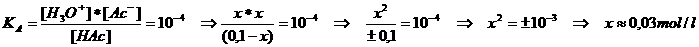We know that HAc is a weak acid. The value of x will be small compared to the [HAc]
or: x can be neglected.
So: 0.03 mol HAc dissociated into ions   → [H3O+] = 0.03 = 3 x 10-2 mol/l   →   pH = 2-log3 = 1.5

Question 47
Never forget:
The weaker an acid, the smaller KA       The stronger an acid, the bigger KA
Check this statement in table I.

Question 48
Explain why the strong acids and bases in the table do not have a K-value.

## 9. Acid base titrations

Demonstration:
On youtube you can find lots of examples of titrations, like the automatic one here.

Note that many of these demonstration films on youtube are bad ones. And don't forget to consult Wikipedia.
In another module we come back to the titrations.
I prefer to use an electronic pH-meter, but doing it with an indicator also is a very good idea.
With a pH-meter you write the pH-value every time after adding, for example, 0.5 ml of the titrant, until reaching and passing the endpoint.
After that, I would demonstrate the same titration without pH-meter, but in the presence of an indicator, knowing already the whereabouts of the endpoint. I am prepared to stop at the very right moment.

Question 49
25 ml xM KOH is titrated with 16 ml 0.27M HCl.
Calculate [KOH].

A titration is a rather quick method to determine the concentration of certain, in water dissolved substances, where apparatus are applied that can read very precisely the volumes of solutions, using pipettes, burettes and graduated cylinders etc.
Another word for titration could be: volumetry

The fundament of an acid base titration is of course the acid base reactionl. An acid an a base react in a fixed mol proportion.
As soon as the two have neutralised each other, you joined 'equivalent' amounts (and you reached the equivalence point).

Question 50
What is the equivalent amount NaOH for 0.3 mol sulfuric acid?

Normally, two dissolved substances react quietly in a titration until the reaction stops: at the moment that equivalent amounts are joined.
In practice - unfortunately - it is quite impossible to stop exactly at the moment that the equivalence point is reached. Mostly we add just a bit too much, we pass that point. Just the last drop will contain a bit too much.
In other words: the endpoint of the titration very often will pass the equivalence point. Always there will be added just a bit too much of the titrant (from the burette), never more than one drop (if you work well).
Officially, the error may not exceed 0.5%.

The indicator of course is our big helper to know when to stop. Att.: chosing the right indicator is not always that easy.

Question 51
In a titration we needed 3 ml of the titrant (from the burette) = about 60 drops. Suppose we added half a drop too much to see the color change, is this titration accurate enough?

At simple titrations, the concentration of the titrant is well known. In that way we can calculate the concentration of the unknown solution.

More about titrations and its applications can be found in another module (14, Quantitative analysis).

Question 52
In a titration of a solution of xM HNO3, we needed 19.87 ml 0,0978M NaOH.
The molarity of the HNO3-solution is close to 0.2M, but we don't know that exactly.
Calculate the exact molarity.

In an acid base titratin, the pH of the equivalence point not always will be 7. The pH dependson the properties of the product (mostly a salt). The product can be built up of particles having an acid or basic character.

For example: if the product is Sodium acetate (a salt), the Sodium ions do not influence the pH, but the acetate ions do. Acetate is a weak base and so the final solution - after the titration of HAc met NaOH (with NaAc as product) - will have a basic environment.
The titration will stop at a pH>7.

We can state the next general rules:
• titrations of a strong acid with a strong base will have a final pH = 7
• titrations of a strong acid with a weak base will have a final pH < 7
• titrations of a weak acid with a strong base will have a final pH > 7

Question 53
Explain why the final pH of the titration of acetic acid with NaOH(aq) will be larger than 7.

Now we hope that it will be clear to you that the choice of indicator for any titration is very important and depends on the kind of titration: You must always check if the product causes a neutral, an acid of a basic solution, and chose an indicator that will change color at the right moment.

Question 54
Is methyl orange suitable as indicator in a titration of HAc with NaOH?

We also know special titrations:
For example, if direct determination of a concenctration is not possible. The substance to investigate could be a gas, or an insoluble solid, or it might be unstable. What to do then?
It is possible to execute an indirect titration.
The substance to determine must first completely react with a "substitute".
• You know exactly how much substitute you first had.
• In a titration you determines exactly how much substitute was left over after the first reaction.
• Then you can calculate how much of the original substance there was.

An example:
Marble contains a high amount of Calcium carbonate. that is unsoluble in water and cannot as such be titrated directly.
We can do now the following: you weigh an amount of marble very precisely. Later, after calculating how much calcium carbonate was in the marbel, you know the percentage. But how to do this in practice?
You bring the weighed amount of marble in an exactly known amount (excess, more then enough) strong acid solution of which you know well the concentration.
All calcium carbonate in the marble will react with the acid until finishing. A certain amount of the acid will be left over.
With a titration you determine now how much acid was left over. That you subtract from the original amount of acid, and you know the amount of acid that reacted with the calcium carbonate.
You know the reaction equation of the acid with calcium carbonate, and thus the mol proportion.
So now you can execute the calculation, find the amount of Calcium carbonate and the percentage of it in the marble.

Question 55
Exactly 10 grammes of marble was dissolved in 150 ml 1M HNO3. A gas was formed and removed by heating.
After that the left over of the nitric acid was titrated with 10 ml 0.2M NaOH.
Calculate the percentage of Carbonate in Marble.

### 9.1 Acid base indicators

The indicators in table VIII are all acid base indicators.
We also know redox-indicators to be used in redox titrations (other module).

The acid base indicator mostly is a weak organic acid with a rather complex structure. Abbreviated formula: HIn.
In water:
HIn + H2OH3O+ + In-       (evenwicht IND)
HIn has color 1         In- has color 2

Imagine this indicator in acid environment, for example in a solution of hydrochloric acid.
In this environment dominate the (H3O+)-ions and the equilibrium IND dislocates to the left. You can also say that in acid environment the indicator takes the HIn performance; color 1 dominates.

Question 56
Explain in your own words what the situation is of an indicator in basic environment.

Every indicator has a zone of change that can be found in the table.
An example: Methyl orange has a change zone of 3.1 - 4.4 (red - orange)
Imagine a strong acid solution with pH=1. Add some drops of Methyl orange solution, that gets the color red.
Now we add strong base like (KOH(aq), the acid will bit by bit be neutralised, the pH-value will gradually increase. It started with pH=1, slowly this value increases.
As soon as the value passes 3.1, then you start to see a color change from red to orange. Only after reaching 4.4 the color has definitly become orange.
Within the change zone, the color is a mix of the two colors.

Question 57

"In the change zone of the indicator the equilibrium is not shifted very much to left, nor to right."

Again: the color of the indicator depends on the environment of the solution.
In acid environment the equilibrium IND dominates at left, as also does the color of the HIn molecules.
Note that the change zone certainly not always is close to 7!

Question 58
Phenolphtaleine and Methyl orange are acid base indicators, but one is less weak than the other.

Question 59
1. What color gets Thymol blue in a solution of 1M KOH?
2. What will dominate in the solution: the HIn molecule or the ion In-?

#### ACTION TASK acid base reactions

 The objective is that you investigate a number of acid base reactions, responding the 8 action points below. If possible you receive in a lab the needed substances. If not, you make the experiment only in your brain. After every observation you must try to draw a (prelimanary) conclusion. The 8 action poinst for every reaction are: The reaction to investigate are: Which particles are present as reactants? (ionic formulas, molecular formulas, structural formulas) Which ones of those particles are the strongest present acid and base, and to what category do they belong? Investigate the solutions with litmus paper and with universal indicator paper or with a pH meter. Write down the read pH. Att.:put the acid in a large test tube. Write down the reactions of the protolysis of the acid and of the base. If the acid or the base can donate more than one proton, then write more protolysis reactions. Write down the total reaction equation, as much as possible with ionic formulas. At which side stands the equilibrium, left or right? Write down the formulas of the products. (ionic, molecular and structural formulas) Add some drops of indicator to the acid and next, add bit by bit the base. Write down the observations and check possible similarities with the total reaction en with the table. Are there follow up reactions? if yes, write them. Oxalic acid(aq) + Lime water 1M hydrochloric acid + Iron(II)sulfide (safe place) Aluminium sulfate + 1M Sodium hydroxyde(aq) Phosphoric acid(aq) + chalk(s) Ammonium chloride (aq) + Potassium hydroxyde respirated air: blow that for some time via a straw in Lime water.

Example:
As an example we chose the first of the above reactions: oxalic acid(aq) + lime water.
Att.: we deal with two solutions to be joined.
Which particles are present as reactants? (ionic formulas, molecular formulas, structural formulas)
 oxalic acid (aq) lime water ionic formulas 2H+ and C2O42- Ca2+ and OH- molecular formulas H2C2O4 Ca(OH)2
structural formulas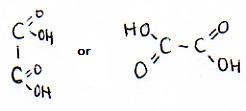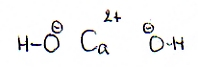Which ones of those particles are the strongest present acid and base, and to what category do they belong?

strongest present acid is the oxalic acid H2C2O4
strongest present base must be: OH- Investigate the solutions with litmus paper and with universal indicator paper or with a pH meter. Write down the read pH. Att.:put the acid in a large test tube.

In practice we find: the acid solution gets a red color with litmus, and the lime water becomes blue.
The other data can only be read with the appropriate apparatus. Write down the reactions of the protolysis of the acid and of the base. If the acid or the base can donate or receive more than one proton, then write more protolysis reactions.
acid:
H2C2O42H+ + C2O42-         H2C2O4H+ + HC2O4-         HC2O4-H+ + C2O42-

base:
OH- + H+H2O Write down the total reaction equation, as much as possible with ionic formulas. At which side stands the equilibrium, left or right?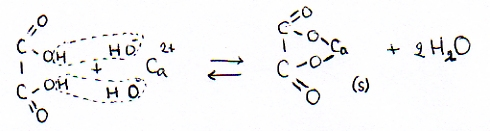just because a precipatiate is formed (the salt Calcium oxalate) the product disappear from the equilibrium. Or: the equilibrium will shift totally to the right, to the products. Write down the formulas of the products. (ionic, molecular and structural formulas)
 Calcium oxalate(s)     en     water ionic formulas Ca2+ en C2O42- (in een ionrooster) molecular formulas Ca2C2O4(s)    en    H2O
structural formulas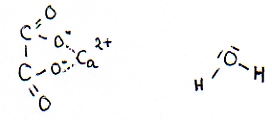Add some drops of indicator to the acid and next, add bit by bit the base.
Write down the observations and check possible similarities with the total reaction en with the table.

Can only be don in the lab. But you will see that every time there is a color change. Are there follow up reactions? if yes, write them down.
First oxalate ions and water are formed, but immediately must be clear that Calcium ions (of the lime) with the oxalate ions produce a bad soluble salt; so there must be some precipitation to be observed. A solid is formed. the solution becomes white trouble.

 Make your choice; chose two substances that toghether can make a good buffer. We chose, for example, ammonium chloride and ammonia. Or: we join NH4+ions and NH3molecules. Now we have a conjugated acid base couple. the ammonium ion is the weak acid and the ammonia is the weak (conjugated) base. Prepare the buffer in a total volume of about 100 ml, and with a buffer capacity of 1 mol/l. 1 mol per liter means in practice about 0.1 mol per 0.1 liter (-100ml) So we must joint: 0.1 mol ammonium chloride and 0.1 mol ammonia in water up to a final volume of 100 ml. You can do this in a graduated volumetric flask of 100 ml that already contained some distilled water. After adding the two substances, you fill the flask exactly up to the marcation of 100 ml. Calculate the pH with the bufferformula and check your calculation by measuring that pH with a pH meter of with pH paper. De buffer formula: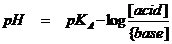The weak acid is ammonium ion which has a pKA (see table) of about 10 the concentrations of acid and base are equal: 0,1mol/l. You may fill that in in the buffer formula You get then: pH = 10 - log 0,1/0,1 = 10 Divide the buffer in two parts of about 50 ml. Add some drops of strong acid to: 50 ml tapwater (knowing its pH) 50 ml buffer Measure of both solutions (so after adding the strong acid) the pH values with pH indicator paper. This can only be done in practice. you can expect the following: Adding some drops of strong acid (like HCl) to distilled water immediately will cause a big pH decrease. The distilled water might have a pH of about 6, and immediately after adding of the acid, that pH can drop to 2. However, if you add the same acid to the buffer with pH 10, that value will hardly drop. Maybe from 10 to 9.9 Now add some drops of strong base to: 50 ml tapwater 50 ml buffer Measure of both solutions (so after adding the strong base) the pH values with pH indicator paper. This can only be done in practice. you can expect the following: Adding some drops of strong base (like NaOH) to distilled water immediately will cause a big pH increase. The distilled water might have a pH of about 6, and immediately after adding of the base, that pH can raise to 10. However, if you add the same base to the buffer with pH 10, that value will hardly raise. Maybe from 10 to 10.1